Search by Topic

Resources tagged with Physics similar to Tangled Trig Graphs:

Filter by: Content type:
Age range:
Challenge level:

There are 78 results

Broad Topics > Applications > PhysicsWhose Line Graph Is it Anyway?

Age 16 to 18 Challenge Level:

Which line graph, equations and physical processes go together?Big and Small Numbers in Chemistry

Age 14 to 16 Challenge Level:

Get some practice using big and small numbers in chemistry.Big and Small Numbers in Physics

Age 14 to 16 Challenge Level:

Work out the numerical values for these physical quantities.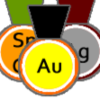Alternative Record Book

Age 14 to 18 Challenge Level:

In which Olympic event does a human travel fastest? Decide which events to include in your Alternative Record Book.Construct the Solar System

Age 14 to 18 Challenge Level:

Make an accurate diagram of the solar system and explore the concept of a grand conjunction.Motion Capture

Age 11 to 16 Challenge Level:

Explore displacement/time and velocity/time graphs with this mouse motion sensor.Escape from Planet Earth

Age 16 to 18 Challenge Level:

How fast would you have to throw a ball upwards so that it would never land?Universal Time, Mass, Length

Age 16 to 18 Short Challenge Level:

Can you work out the natural time scale for the universe?Ramping it Up

Age 16 to 18 Challenge Level:

Look at the calculus behind the simple act of a car going over a step.The Not-so-simple Pendulum 1

Age 16 to 18 Challenge Level:

See how the motion of the simple pendulum is not-so-simple after all.Perfect Eclipse

Age 14 to 16 Challenge Level:

Use trigonometry to determine whether solar eclipses on earth can be perfect.Ideal Gases

Age 16 to 18 Challenge Level:

Problems which make you think about the kinetic ideas underlying the ideal gas laws.Ideal Axes

Age 16 to 18 Challenge Level:

Explore how can changing the axes for a plot of an equation can lead to different shaped graphs emergingDiamonds Aren't Forever

Age 16 to 18 Challenge Level:

Ever wondered what it would be like to vaporise a diamond? Find out inside...The Lorentz Force Law

Age 16 to 18 Challenge Level:

Explore the Lorentz force law for charges moving in different ways.Approximately Certain

Age 14 to 18 Challenge Level:

Estimate these curious quantities sufficiently accurately that you can rank them in order of sizeConstantly Changing

Age 14 to 16 Challenge Level:

Many physical constants are only known to a certain accuracy. Explore the numerical error bounds in the mass of water and its constituents.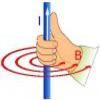Electromagnetism

Age 16 to 18Powerfully Fast

Age 16 to 18 Challenge Level:

Explore the power of aeroplanes, spaceships and horses.Reaction Types

Age 16 to 18 Challenge Level:

Explore the rates of growth of the sorts of simple polynomials often used in mathematical modelling.Levels of Bohr

Age 16 to 18 Challenge Level:

Look at the units in the expression for the energy levels of the electrons in a hydrogen atom according to the Bohr model.The Power of Dimensional Analysis

Age 14 to 18

An introduction to a useful tool to check the validity of an equation.Which Twin Is Older?

Age 16 to 18

A simplified account of special relativity and the twins paradox.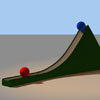Whoosh

Age 16 to 18 Challenge Level:

A ball whooshes down a slide and hits another ball which flies off the slide horizontally as a projectile. How far does it go?Moving Stonehenge

Age 16 to 18 Challenge Level:

A look at the fluid mechanics questions that are raised by the Stonehenge 'bluestones'.Resistance

Age 16 to 18 Challenge Level:

Find the equation from which to calculate the resistance of an infinite network of resistances.Earth Orbit

Age 16 to 18 Challenge Level:

Follow in the steps of Newton and find the path that the earth follows around the sun.Cannon Balls

Age 16 to 18 Short Challenge Level:

How high will a ball taking a million seconds to fall travel?Mach Attack

Age 16 to 18 Challenge Level:

Have you got the Mach knack? Discover the mathematics behind exceeding the sound barrier.Pumping the Power

Age 16 to 18 Challenge Level:

What is an AC voltage? How much power does an AC power source supply?Gravity Paths

Age 16 to 18 Challenge Level:

Where will the spaceman go when he falls through these strange planetary systems?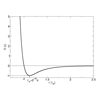Lennard Jones Potential

Age 16 to 18 Challenge Level:

Investigate why the Lennard-Jones potential gives a good approximate explanation for the behaviour of atoms at close rangesStriking Gold

Age 16 to 18 Challenge Level:

Investigate some of the issues raised by Geiger and Marsden's famous scattering experiment in which they fired alpha particles at a sheet of gold.Lunar Leaper

Age 16 to 18 Challenge Level:

Gravity on the Moon is about 1/6th that on the Earth. A pole-vaulter 2 metres tall can clear a 5 metres pole on the Earth. How high a pole could he clear on the Moon?Cobalt Decay

Age 16 to 18 Challenge Level:

Investigate the effects of the half-lifes of the isotopes of cobalt on the mass of a mystery lump of the element.Age 16 to 18 Challenge Level:

A look at a fluid mechanics technique called the Steady Flow Momentum Equation.Big and Small Numbers in Physics - Group Task

Age 16 to 18 Challenge Level:

Work in groups to try to create the best approximations to these physical quantities.The Real Hydrogen Atom

Age 16 to 18 Challenge Level:

Dip your toe into the world of quantum mechanics by looking at the Schrodinger equation for hydrogen atomsStemnrich - Technology

Age 11 to 16 Challenge Level:

This is the technology section of stemNRICH - Core.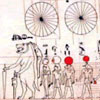Ancient Astronomical Terms

Age 11 to 18

Some explanations of basic terms and some phenomena discovered by ancient astronomers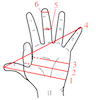Age 14 to 16 Challenge Level:

Which units would you choose best to fit these situations?New Units for Old

Age 16 to 18 Challenge Level:

Can you match up the entries from this table of units?A Question of Scale

Age 14 to 16 Challenge Level:

Use your skill and knowledge to place various scientific lengths in order of size. Can you judge the length of objects with sizes ranging from 1 Angstrom to 1 million km with no wrong attempts?Go Spaceship Go

Age 16 to 18 Challenge Level:

Show that even a very powerful spaceship would eventually run out of overtaking powerDrug Stabiliser

Age 16 to 18 Challenge Level:

How does the half-life of a drug affect the build up of medication in the body over time?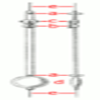The Not-so-simple Pendulum 2

Age 16 to 18 Challenge Level:

Things are roughened up and friction is now added to the approximate simple pendulumThe Ultra Particle

Age 16 to 18 Challenge Level:

Explore the energy of this incredibly energetic particle which struck Earth on October 15th 1991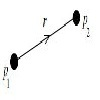Age 16 to 18 Challenge Level:

Can you arrange a set of charged particles so that none of them start to move when released from rest?Modelling Assumptions in Mechanics

Age 16 to 18

An article demonstrating mathematically how various physical modelling assumptions affect the solution to the seemingly simple problem of the projectile.Eudiometry

Age 16 to 18 Challenge Level:

When a mixture of gases burn, will the volume change?